### 404页面的识别

#### 列举几种可能遇到的情况

##### 状态码=404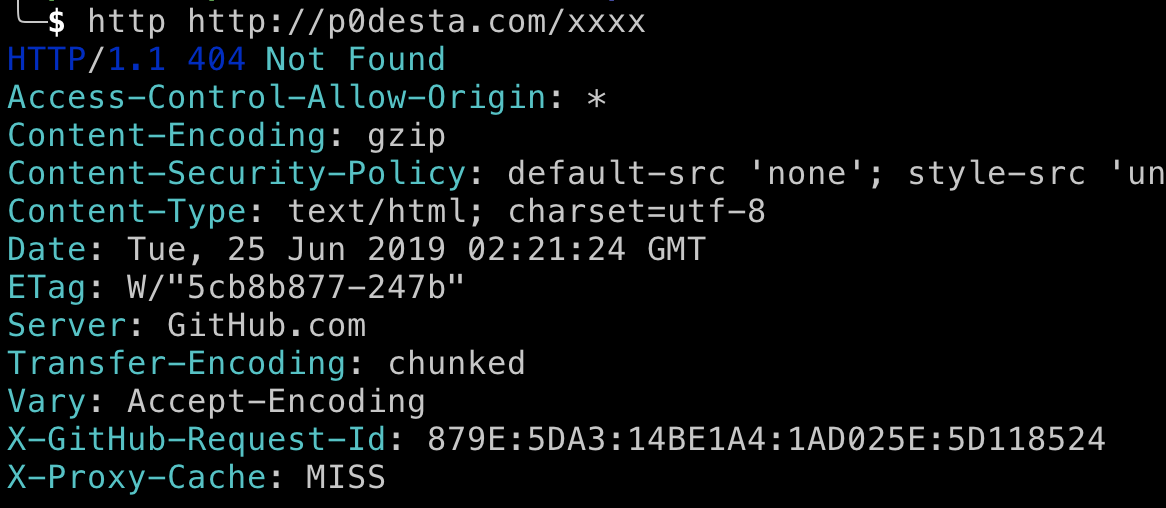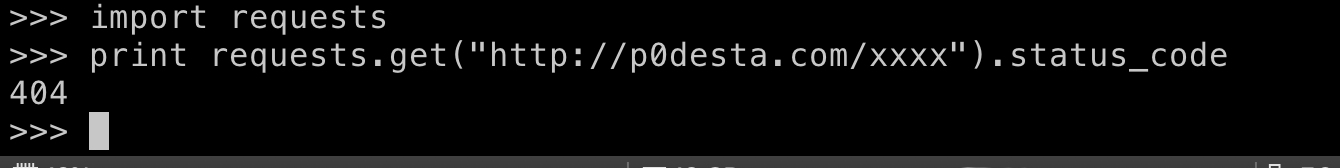##### 跳转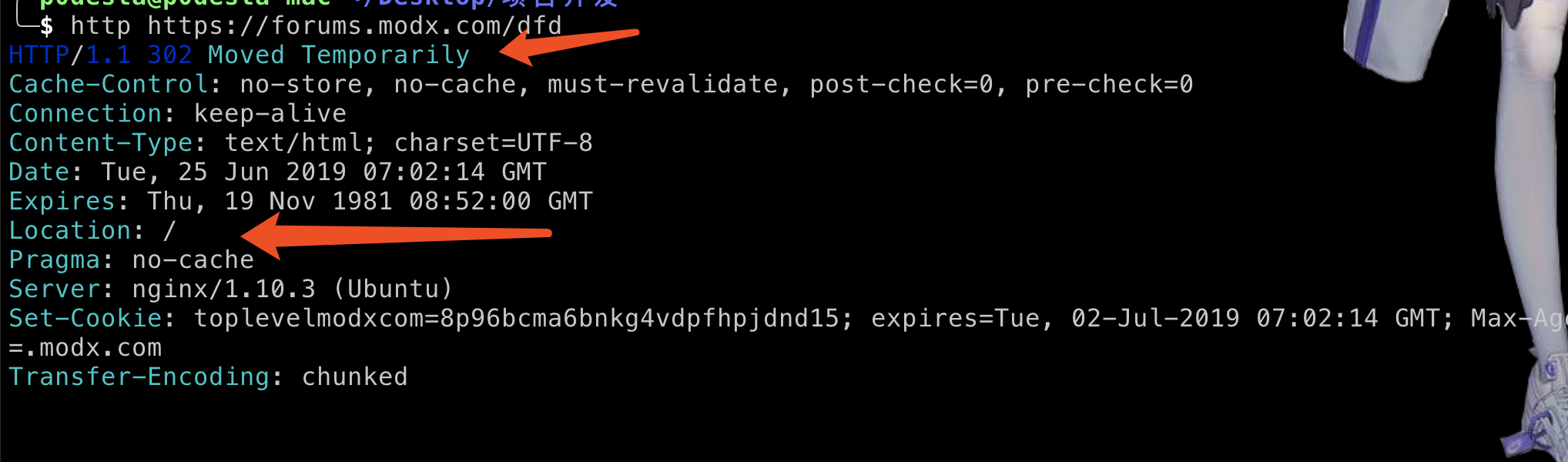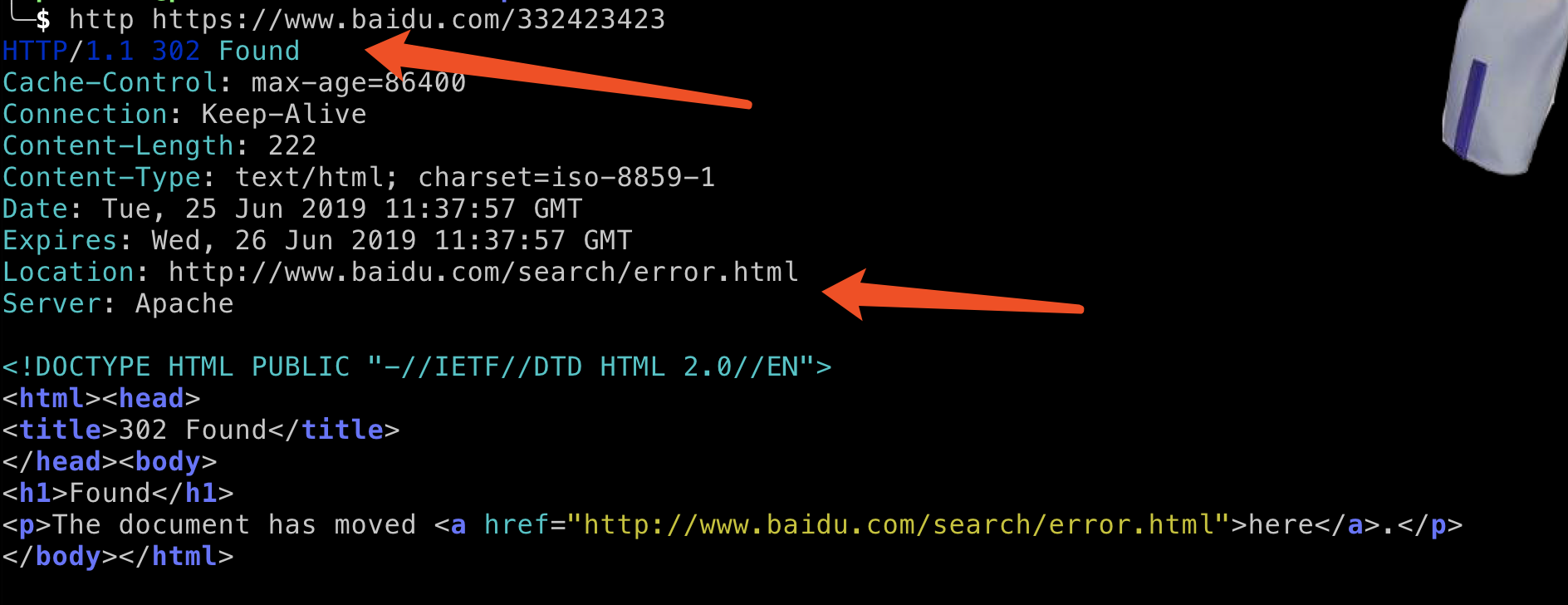##### 不跳转、显示报错页面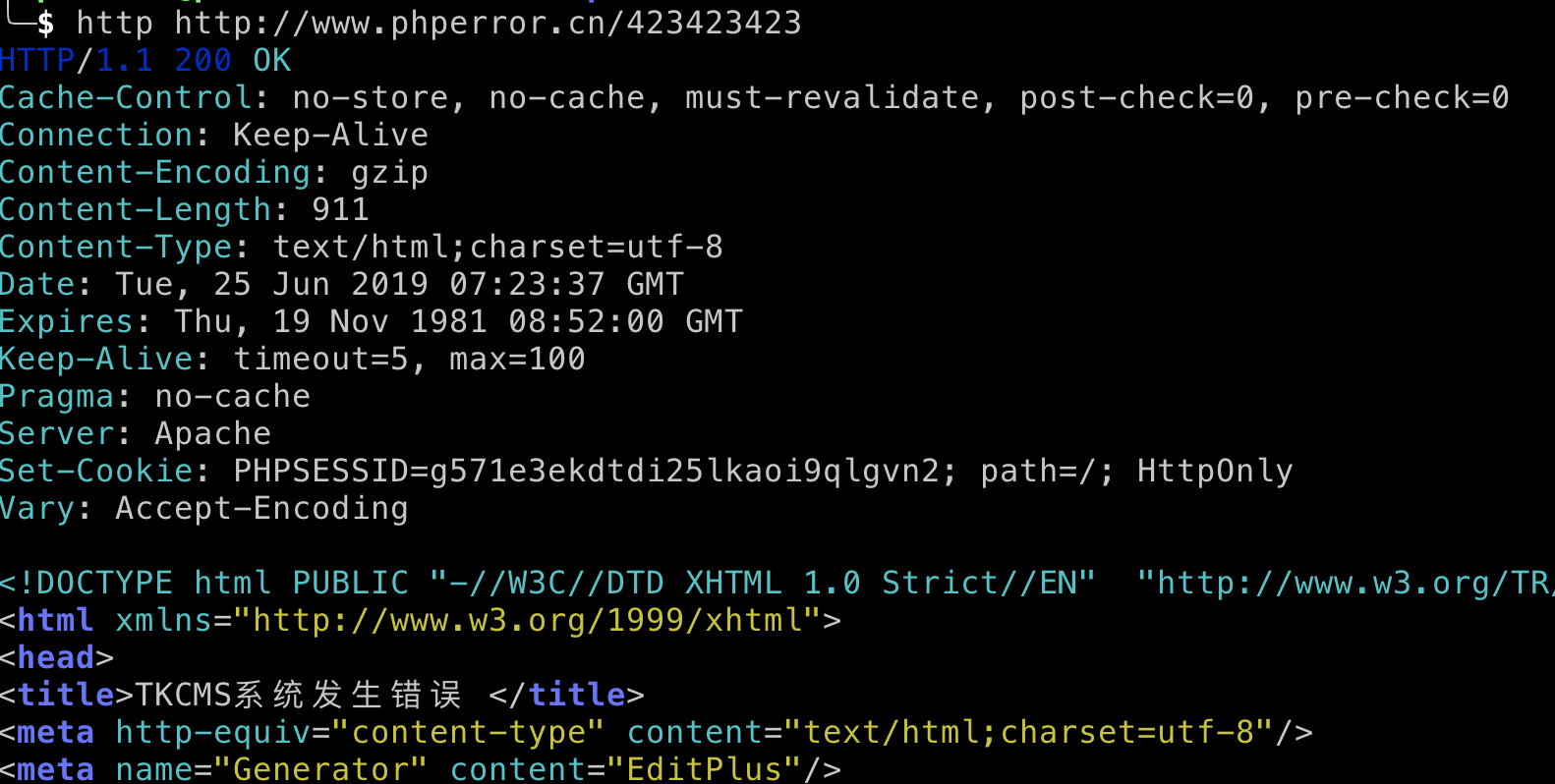#### 解决方法

​ 首先在<<白帽子讲web扫描>>中给的方法是,通过状态码和页面内容两个维度去分析,比如说我们可以构造类似no_exists_for_test.html这种情况的页面来触发404,在实际的文件名构造中可以加入随机因子,然后将这些页面的特征进行提取和储存,当访问一个页面的时候先判断状态码是否为404,如果不是的话再去与404页面进行相似度的比较,如果高于阀值的话就判定为404页面。

• SimHash

• 余弦相似性

这个计算方法是根据余弦的夹角来判断相似性的,因为一开始我们需要提取数据然后进行向量化,比如说向量化后的数据为A=[x1, y1],B=[x2, y2],那么通过公式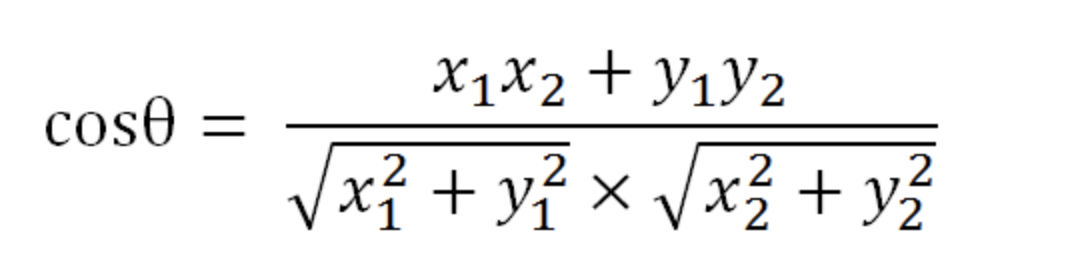我们可以计算出角度,那么我们可以通过夹角的大小，来判断向量的相似程度。夹角越小，就代表越相似。

有人会问这是两个点的坐标来计算的,网页提取完肯定不会是两个点啊,当然,余弦的这种计算方法对n维向量也成立,那么我们可以使用这个公式来计算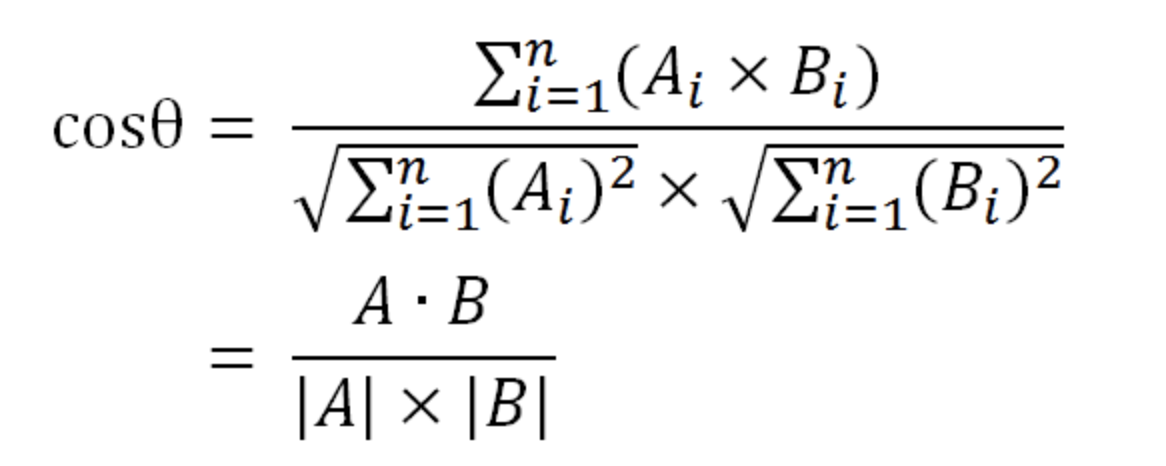• 皮尔逊相关系数

皮尔逊相关系数是余弦相似度在维度缺失的情况下的一种改进,为什么这么说呢,如果对于如下这种向量

``````v1 = (1, 2, 4),

v2=(3, -1, null)``````

因为这两个向量由于v2中第三个维度有null, 无法使用余弦相似性来计算,皮尔逊相关系数公式实际上就是在计算夹角余弦之前将两个向量减去各个样本的平均值，达到中心化的目的。从知友的回答可以明白，皮尔逊相关函数是余弦相似度在维度缺失上面的一种改进方法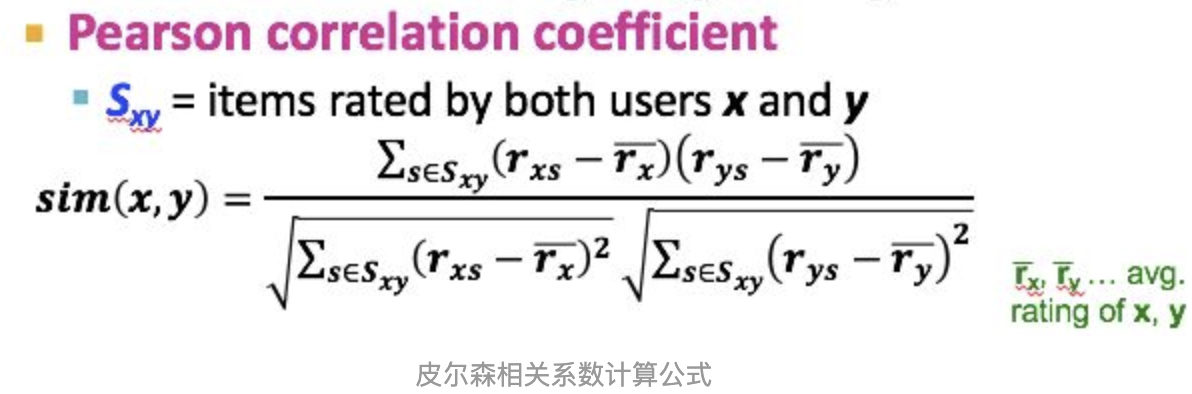```class TextExtraction:
def __init__(self, url, number):
self.url = url

def text_extraction(self):
try:
resp = requests.get(url=self.url, timeout=1)
except BaseException as e:
raise e
resp.encoding='utf-8'
soup = BeautifulSoup(resp.text,'lxml')
title_text = soup.title.string
body_text = soup.find("body").get_text().replace("\n", "").replace("\r", "").replace("\t", "").replace(" ", "")
text = title_text + body_text
return text

class Similarity:
def __init__(self, target1, target2, topK=30):
self.target1 = target1
self.target2 = target2
self.topK = topK

def vector(self):
self.vdict1 = {}
self.vdict2 = {}
top_keywords1 = jieba.analyse.extract_tags(self.target1, topK=self.topK, withWeight=True)
top_keywords2 = jieba.analyse.extract_tags(self.target2, topK=self.topK, withWeight=True)
for k, v in top_keywords1:
self.vdict1[k] = v
for k, v in top_keywords2:
self.vdict2[k] = v

def mix(self):
for key in self.vdict1:
self.vdict2[key] = self.vdict2.get(key, 0)
for key in self.vdict2:
self.vdict1[key] = self.vdict1.get(key, 0)

def mapminmax(vdict):
_min = min(vdict.values())
_max = max(vdict.values())
_mid = _max - _min
for key in vdict:
vdict[key] = (vdict[key] - _min)/_mid
return vdict

self.vdict1 = mapminmax(self.vdict1)
self.vdict2 = mapminmax(self.vdict2)

def similar(self):
self.vector()
self.mix()
sum = 0
for key in self.vdict1:
sum += self.vdict1[key] * self.vdict2[key]
A = sqrt(reduce(lambda x,y: x+y, map(lambda x: x*x, self.vdict1.values())))
B = sqrt(reduce(lambda x,y: x+y, map(lambda x: x*x, self.vdict2.values())))
return sum/(A*B)
```

``````https://thief.one/2018/04/12/1/
https://www.cnblogs.com/shaosks/p/9121774.html
https://zhuanlan.zhihu.com/p/33164335``````